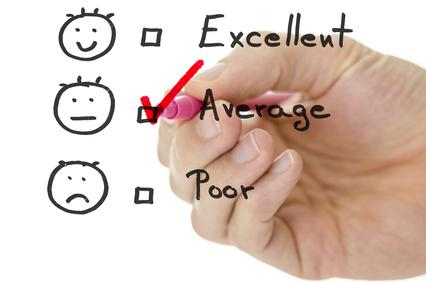# Average Value & Calculation

The average is the middle value calculated by adding up all the numbers and then dividing the result by the total quantity of figures that were added. In this article, we are going to discuss the definition of an average and how to calculate the average for the given data with more examples.How many of you have been called average by the teachers in your class or by friends from your sports club? Most of us. So when is this average word used and what is its meaning?

Sometimes, to generalize the information we obtain an average value so that the calculation becomes easy and understandable. Do you have a fair idea of the average height of students in your class? Or average age? You can of course calculate! Let us understand the concept of calculating the average.

## How to Calculate the Average?

An average is a number that we get by adding all the quantities together and then dividing this total by the number of quantities. That’s all about average!

For example: Out of 20 marks, you scored 15 in maths. Your friends had a score of 10 and 5 respectively. The average scores of all three will be the total number of marks all the three scored together, divided by the total number of people that is 3.

So, Average here becomes (15+10+5)/3 which equals 10. Your marks are above average.In our daily lives, we come across the average calculation frequently. Sachin Tendulkar’s average runs in his test matches. Average temperature. The average age of the family. Based on the calculation, we can find who lives longer on an average: men or women. Or on average, people who don’t smoke are healthier than those who do. Numerous problems based on the average is asked in our aptitude exams.

Calculate the Average – Practice Problem

A family consists of two grandparents, two parents, and three children. The average age of the grandparents is 65 years, that of the parents is 35 years, and that of the grandchildren is 5 years. What would be the average age of the family?

The fundamental remains the same. You just need to practice and implement in your word problems. Important questions with necessary explanations are provided on BYJU’S applications. Learn and enjoy!